Mobile QR Code1. (Electronic Engineering Department, Kyung Hee University, Yongin-si, Gyeonggi-do 17104, Korea)

CMOS, phased-array system, phase shifter, switched-network type

## I. INTRODUCTION

A phased-array is an essential technique for recent wireless systems. It can enhance the radiation power to certain direction by beamforming, which is controlled by the phase of each radiated signal from an antenna array. A phase shifter is a core device for the phased-array technique. Hence, the performance of the phased-array is mainly determined by the performance of the phase shifter.

A switched-network type is one of the common configurations for phase shifter design [1-3]. It consists of a couple of phase shift blocks. Each block includes two paths providing different phase shift. The control switches are used to select the right path for the desired phase shift. Using more bits for switching guarantees higher phase resolution at the cost of size increase. Unlike a vector summing type using active devices for phase shifter design [4-6], the switched-network type does not consume DC power.

In this paper, we presented the switched-network type single-pole double-throw CMOS phase shifter for the frequency range including industrial-scientific-medical (ISM) band. Each phase shift block was designed with inductor-capacitor (LC) filters. To achieve compact size, some phase shift block employed an embedded type filter topology sharing inductors for two different relative phase paths.

## II. ANALYSIS AND DESIGN

Fig. 1 shows the full schematic of the 6-bit phase shifter. The switching type is a single-pole-double-throw. Each phase shift block was switched by NMOS transistors with the supply voltage of 1 V. All the switch transistors were optimized to reduce the insertion loss. All the phase shift blocks were designed with conventional LC filter topologies such as low-pass filter (LPF), high-pass filter (HPF), and series/parallel resonator. Specially, the phase shift of 45$^{\circ}$, 22.5$^{\circ}$ and 11.25$^{\circ}$ were designed with the embedded device type . There are 6 bias ports (SW6, SW5, SW4, SW3, SW2, SW1), which correspond to 6-bit code values to control the switches. SW6 is the most significant bit and SW1 is the least significant bit. 64 states (64 relative phase shifts covering 360$^{0}$ with the step of 5.625$^{0}$) were designed by using these 6 bits. The value of all the resistors was chosen to be 10 k${\Omega}$.

##### Fig. 1. Schematic of 6-bit phase shifter.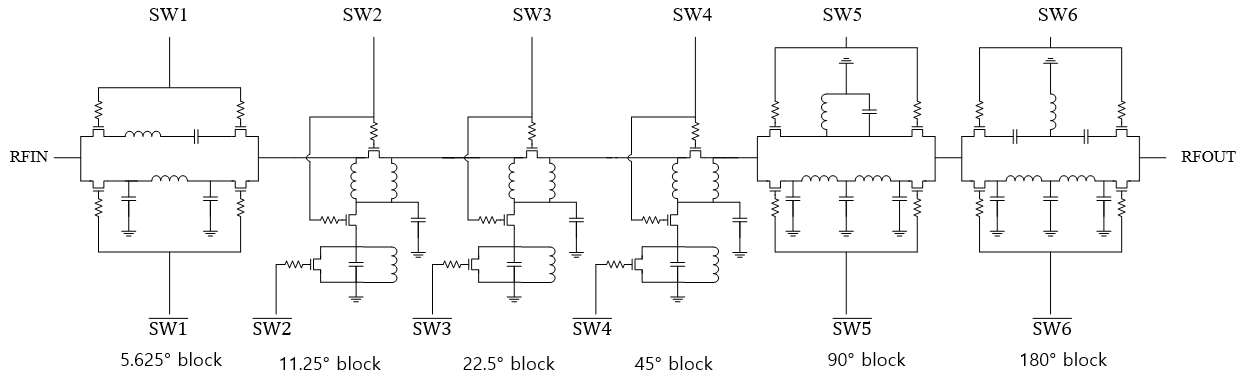### A. 180°, 90° and 5.625° Phase Shift Blocks

The 180$^{\circ}$ phase shift block consisted of LPF and HPF (Fig. 2). Two filters were designed to have 180$^{\circ}$ relative phase difference in the interested frequency range. Inductors and capacitors were optimized to reduce the insertion loss while achieving relative phase shift. For LPF design, the fifth order network was used to reduce the insertion loss.

The 90$^{\circ}$ phase shift block was designed with LPF and parallel resonator (Fig. 3). At the interested frequencies, the ‘0’ state path was designed with a ${\lambda}$/4 transmission line and ‘1’ state path was designed with the parallel LC resonator. Similarly, the fifth order LPF was incorporated.

The 5.625$^{\circ}$ phase shift block is shown in Fig. 4. The LC series resonator and LPF were used. To design this small phase shift, the design technique reported in  was adopted.

##### Fig. 2. Schematic of 180$^{\circ}$ phase shift block (L$_{1}$=3.3 nH, C$_{1}$=1.5 pF, L$_{2}$=2.1 nH, C$_{2}$=0.8 pF, C$_{3}$=1.7 pF).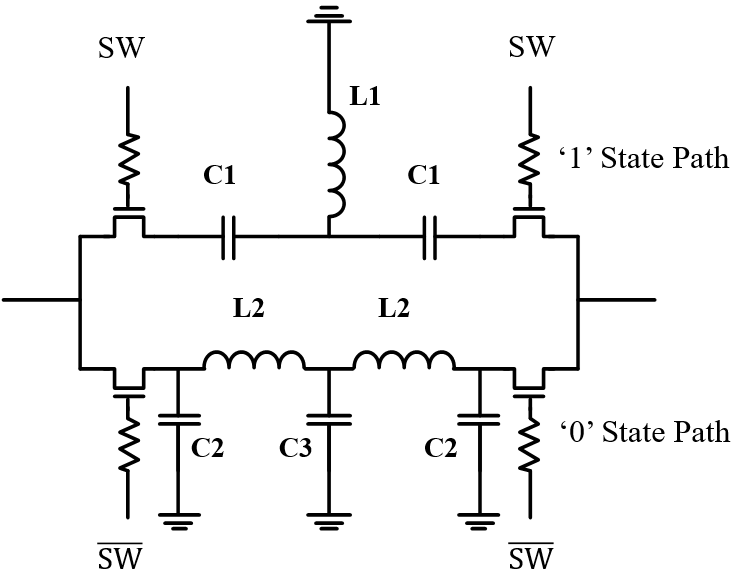##### Fig. 3. Schematic of 90$^{\circ}$ phase shift block (L$_{1}$=2.4 nH, C$_{1}$=1.3 pF, L$_{2}$=2.3 nH, C$_{2}$=0.43 pF, C$_{3}$=1 pF).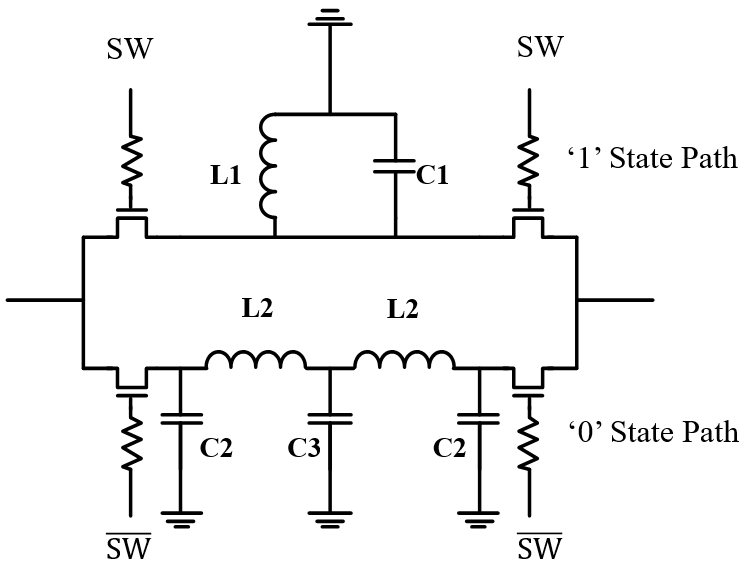##### Fig. 4. Schematic of 5.625$^{\circ}$ phase shift block (L$_{1}$=0.45 nH, C$_{1}$=9.7 pF, L$_{2}$=0.51 nH, C$_{2}$=72 fF).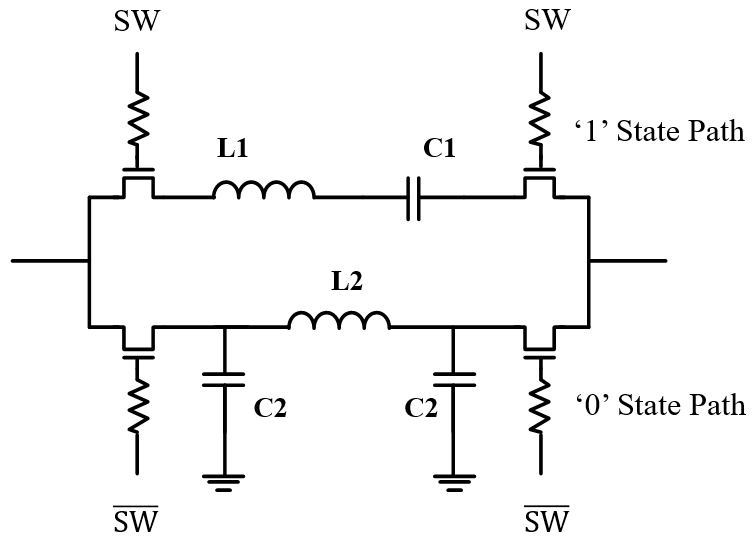### B. 45°, 22.5° and 11.25° Phase Shift blocks

Fig. 5(a) shows the embedded type topology for 45$^{\circ}$, 22.5$^{\circ}$ and 11.25$^{\circ}$. In ‘0’ state, M$_{1}$ and M$_{2}$ are off, and M$_{3}$ is on. The C$_{\mathrm{a}}$ in Fig. 5(b) consists of C$_{1}$ and parasitic capacitances from M$_{2}$. In ‘1’ state, M$_{1}$ and M$_{2}$ are on, and M$_{3}$ is off. The C$_{\mathrm{b}}$ in Fig. 5(c) includes C$_{1}$, C$_{2}$ and parasitic capacitances from M$_{3}$.

L$_{1}$ and C$_{\mathrm{a}}$ were calculated by the following equations. S-parameters were expressed as

##### (1)
$S_{11}=\frac{j\left(2\omega L_{1}-\omega ^{3}{L_{1}}^{2}C_{a}-\omega C_{a}{Z_{0}}^{2}\right)}{2Z_{0}\left(1-\omega ^{2}L_{1}C_{a}\right)+j\left(2\omega L_{1}-\omega ^{3}{L_{1}}^{2}C_{a}+\omega C_{a}{Z_{0}}^{2}\right)}$
##### Fig. 5. (a) Schematic of 45$^{\circ}$ phase shift block (L$_{1}$=1.3 nH, C$_{1}$=0.81 pF, L$_{2}$ =3 nH, C$_{2}$=0.1 pF), 22.5$^{\circ}$ phase shift block (L$_{1}$=0.66 nH, C$_{1}$=0.24 pF, L$_{2}$=4.6 nH, C$_{2}$=63 fF), and 11.25$^{\circ}$ phase shift block (L$_{1}$=0.27 nH, C$_{1}$=10 fF, L$_{2}$=6.5 nH, C$_{2}$=10 fF); (b) equivalent circuit of ‘0’ state; (c) equivalent circuit of ‘1’ state.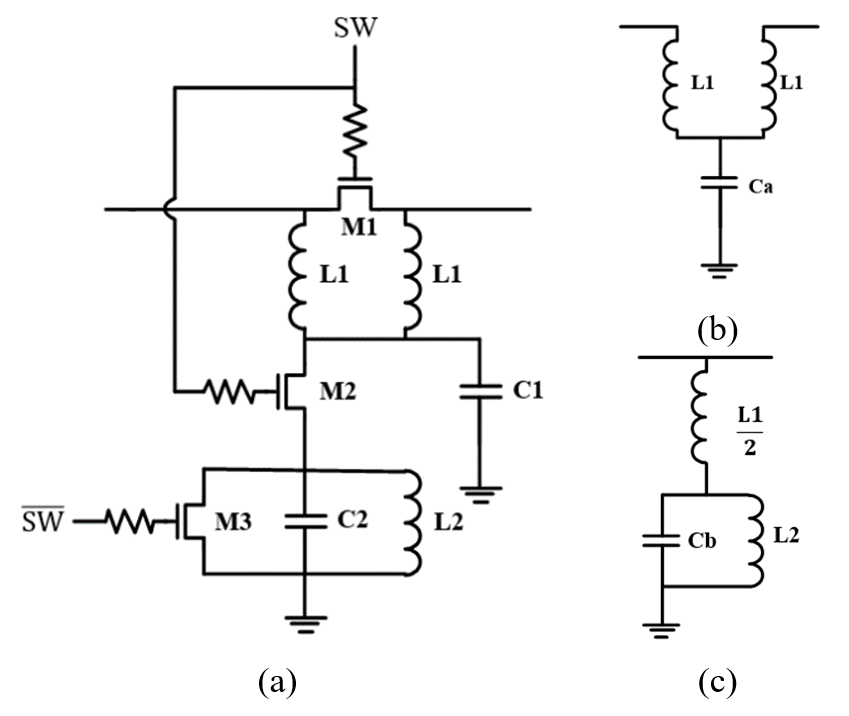##### (2)
$S_{21}=\frac{2Z_{0}(1-\omega ^{2}L_{1}C_{a})+j2(2\omega L_{1}-\omega ^{3}{L_{1}}^{2}C_{a})}{2Z_{0}(1-\omega ^{2}L_{1}C_{a})+j(2\omega L_{1}-\omega ^{3}{L_{1}}^{2}C_{a}+\omega C_{a}{Z_{0}}^{2})}$.

To obtain maximum return loss at the interested frequency, L$_{1}$ and C$_{\mathrm{a}}$ were set as

##### (3)
${\omega _{0}}^{2}=\frac{1}{L_{1}C_{a}}$, $Z_{0}=\sqrt{\frac{L_{1}}{C_{a}}}$.

Then, S$_{21}$ was expressed as

##### (4)
$S_{21}=\frac{2Z_{0}}{2Z_{0}(1-\omega ^{2}L_{1}C_{a})+j(2\omega L_{1}-\omega ^{3}{L_{1}}^{2}C_{a}+\omega C_{a}{Z_{0}}^{2})}$.

##### (6)
$L_{1}=\frac{Z_{0} \tan (\Delta \theta / 2)}{\omega_{0}} \quad C_{a}=\frac{\sin (\Delta \theta)}{Z_{0} \omega_{0}}$

The phase slope with ‘1’ state and ‘0’ state at the operating frequency was derived as

##### (7)
$\frac{\partial }{\partial \omega }\left.\left(-\tan ^{-1}\left(\frac{2\omega L_{1}-\omega ^{3}{L_{1}}^{2}C_{a}+\omega C_{a}{Z_{0}}^{2}}{2Z_{0}\left(1-\omega ^{2}L_{1}C_{a}\right)}\right)\right)\right| _{\omega ={\omega _{0}}}=-\frac{2L_{1}}{Z_{0}}$
##### (8)
$\frac{\partial}{\partial \omega}\left(-\left.\tan ^{-1}\left(\frac{Z_{0}}{\omega\left(L_{1}+\frac{2 L_{2}}{1-\omega^{2} L_{2} C_{b}}\right)}\right)\right|_{\omega=\omega_{0}=\frac{1}{\sqrt{L_{2} C_{b}}}}=-C_{b} Z_{0},\right.$,

respectively. For the wideband performance, the phase slopes need to be same. With the characteristic impedance Z$_{0}$ of 50 ${\Omega}$ and the operating frequency of 2.4 GHz, L$_{2}$ and C$_{\mathrm{b}}$ were calculated as

##### (9)
$L_{2}=\frac{1}{\omega_{0}{ }^{2} C_{b}} \quad C_{b}=\frac{2 L_{1}}{Z_{0}{ }^{2}}=\frac{2 \tan (\Delta \theta / 2)}{Z_{0} \omega_{0}} .$

## III. IMPLEMENTATION AND MEASUREMENT RESULT

The 6-bit phase shifter was implemented using Samsung 65 nm RF CMOS IC process. On chip spiral octagonal inductor and metal-insulator-metal capacitors were used. To reduce loss and size, one center-tap symmetric inductor, which was provided by the design kit, were employed to implement two L$_{1}$ inductors in Fig. 5. Hence, the topology was similar to the structure using magnetic coupling between two inductors in . The switch transistors were large enough to minimize the insertion loss. Fig. 6 shows a photograph of the fabricated phase shifter. The chip was mounted and bondwired on the open face package with 16 leads, sized 4 mm ${\times}$ 4 mm. The total die area including the bonding pads was 1.4 ${\times}$ 0.81 mm$^{2}$. S-parameters were measured using a vector network analyzer (5063A, Keysight). The measured and simulated scattering parameters of the fabricated chip for all 64 states are shown in Fig. 7-13. In the frequency range of 2.12 GHz $-$ 2.51 GHz, the input and output return loss were above 8.2 dB and 10 dB, respectively (Fig. 7-10). Fig. 11 shows the insertion loss of -9.7${\pm}$1.5 dB, which is 0.9 dB lower than simulation results. Fig. 12 shows the phase shift of all 64 states with respect to the reference state. The root-mean-square (rms) phase error at the frequency from 2 GHz to 2.8 GHz is shown in Fig. 13. From 2.12 GHz to 2.51 GHz, the measured rms phase error and rms amplitude error were less than 3$^{\circ}$ and 0.87 dB, respectively. Table 1 shows the comparison of the phase shifter designed in this study to those in the literature. The fabricated phase shifter shows the small rms phase error, and the smallest size.

##### Fig. 6. Photograph of the fabricated 6-bit phase shifter.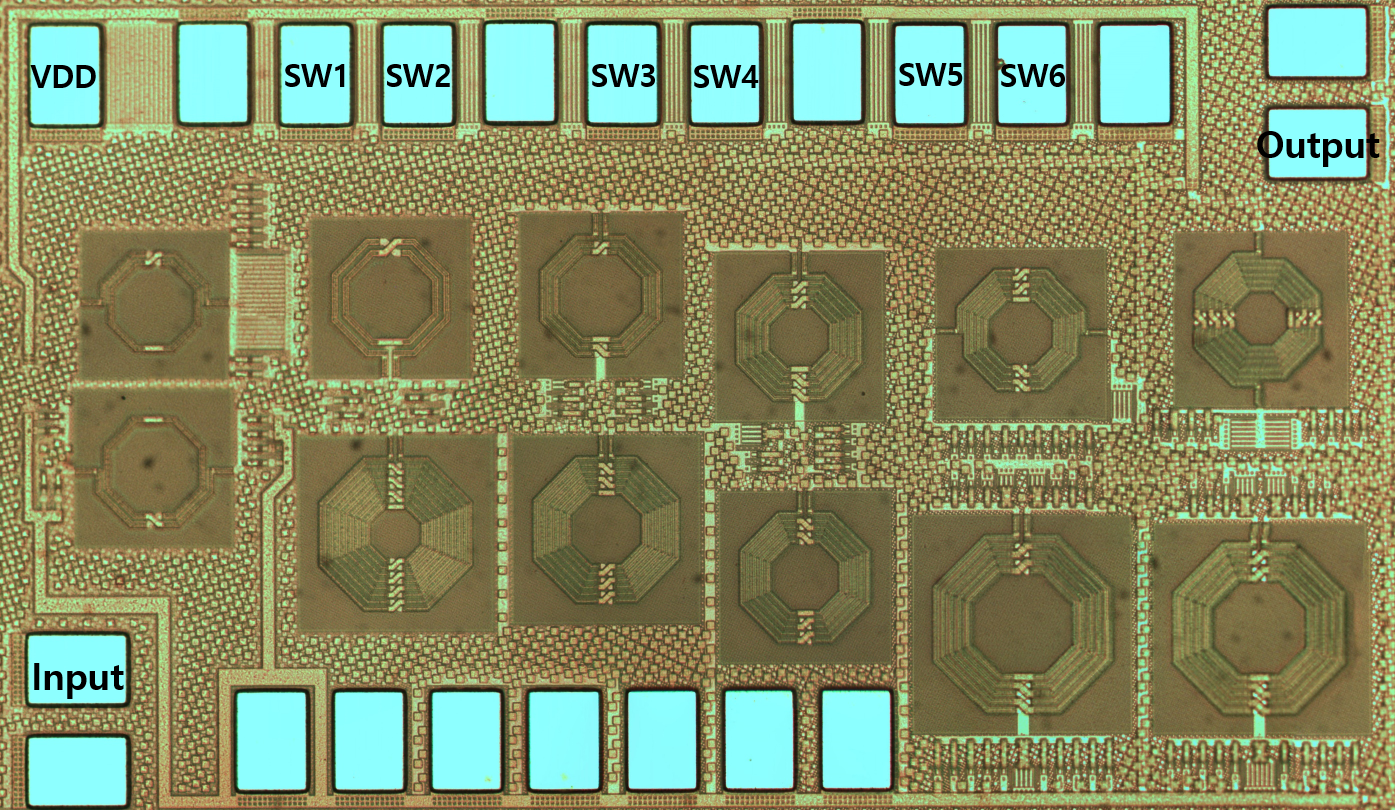##### Fig. 7. Simulation results of input return loss.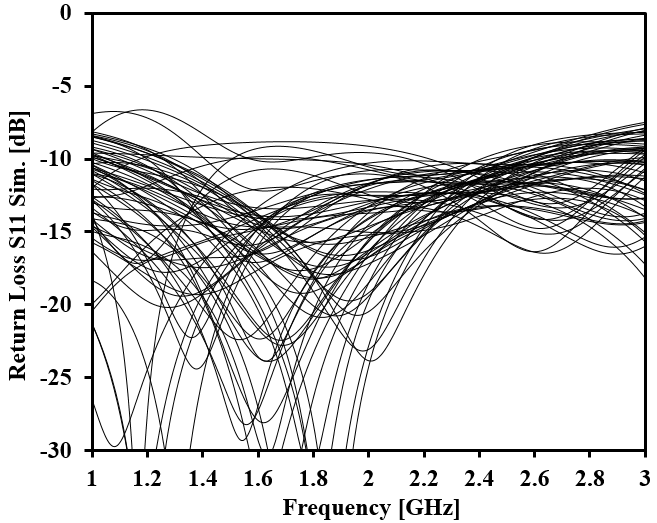##### Fig. 8. Measurement results of input return loss.##### Fig. 9. Simulation results of output return loss.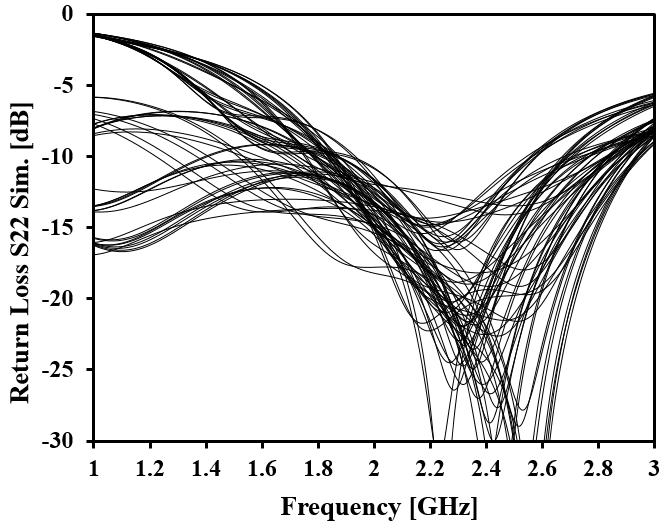##### Fig. 10. Measurement results of output return loss.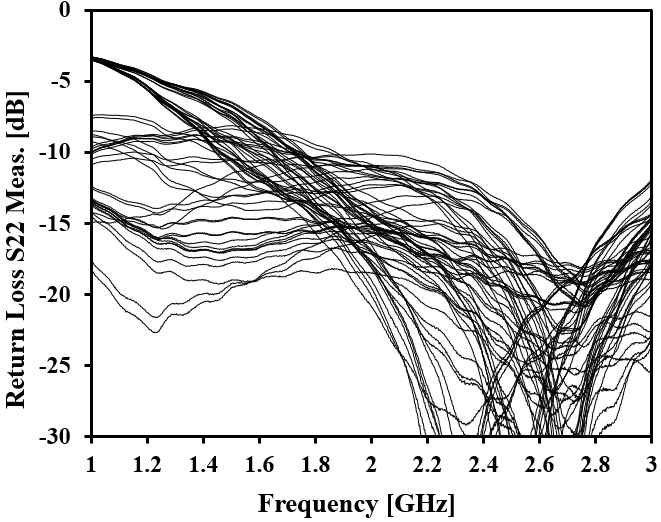##### Fig. 11. Simulation and measurement results of insertion loss.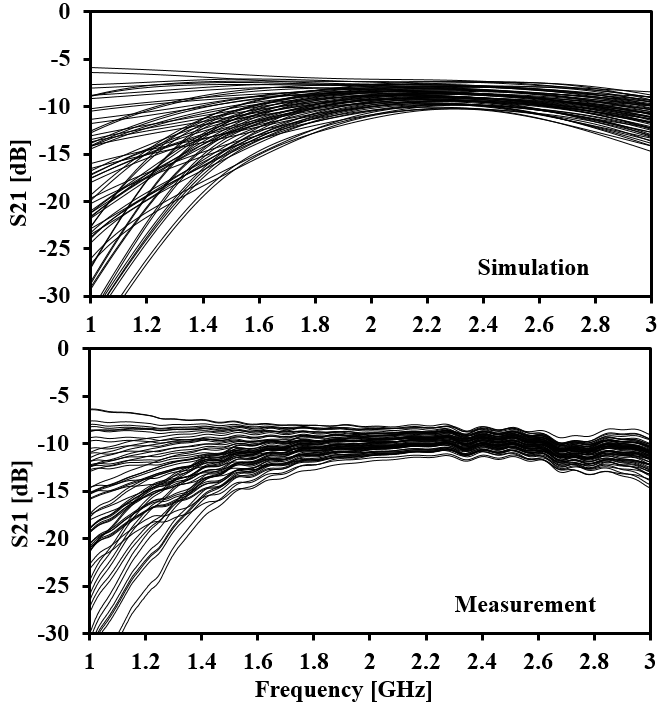##### Fig. 12. Measured phase shift of 64 states.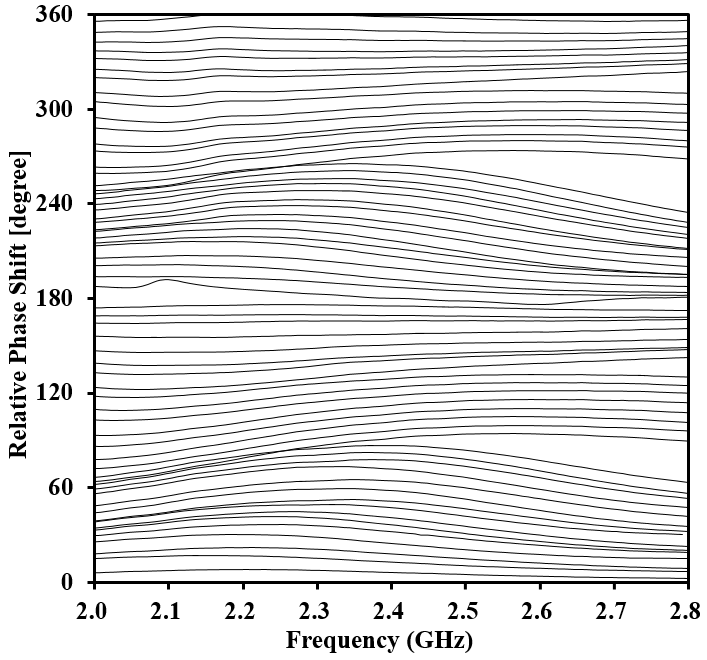##### Fig. 13. Measured and simulated rms phase error.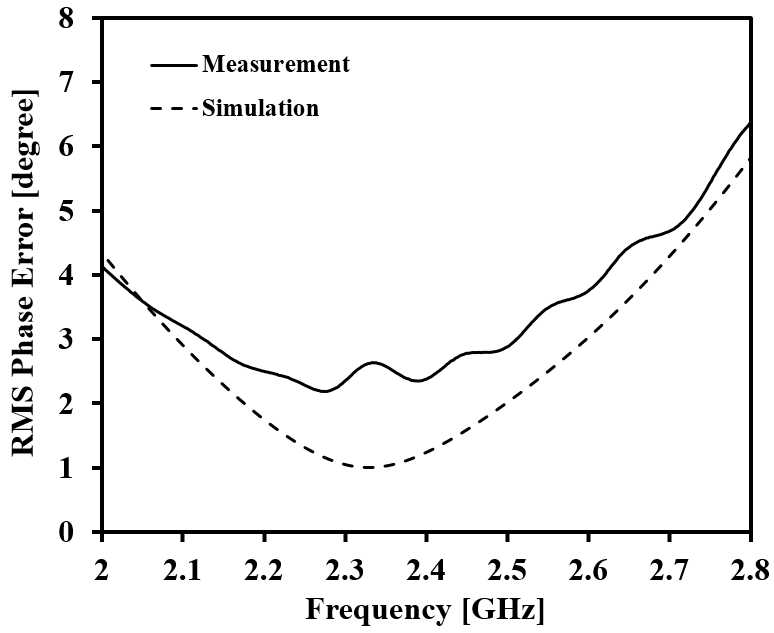##### Table 1. Comparison of Previously Reported 6-Bit Phase Shifters
 Reference   This work Technology 0.5 ${\mu}$m GaAs 0.18 ${\mu}$m CMOS 65 nm CMOS Frequency (GHz) 1.4-2.4 2.5-3.2 2.12-2.51 Gain (dB) -3.8±0.7 -2.5±1.5 -9.7±1.5 RMS phase error (degree) 4° 2° 3° Die area (mm$^{2}$) 3.31 4.16 1.134 DC power (mW) 0 60 0

## IV. CONCLUSION

A 6-bit phase shifter fabricated using 65 nm RF CMOS technology was presented. The phase shifter employed the switched-network topology with LC filter configurations. To reduce the size, the embedded type topology was used for some phase shift blocks. To the best of our knowledge, this was the phase shifter showed the smallest size in comparison with other 6-bit phase shifters for ISM band.

## ACKNOWLEDGMENTS

This work was supported by the National Research Foundation of Korea (NRF) grant funded by the Korea government (MSIT) (No. 2022R1A2C1007712). The chip fabrication and EDA tool were supported by the IC Design Education Center (IDEC), Korea.

## References

1
Xiao Q., Oct. 2011, A Compact L-band broadband 6-bit MMIC phase shifter with low phase error, Eur. Microw. Integr. Circuits Conf., pp. 410-413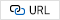2
Meghdadi M., Azizi M., Kiani M., Medi A., Atarodi M., Dec. 2010, A 6-Bit CMOS phase shifter for S - Band, IEEE Trans. Microw. Theory Techn., Vol. 58, No. 12, pp. 3519-35263
Alsuraisry H., Cheng J. H., Wang H. W., Zhong J. Y., Tsai J. H., Huang T. W., Dec. 2015, A X-band digitally controlled 5-bit phase shifter in 0.18-${\mu}$m CMOS technology, Asia-Pacific Microw. Conf.4
Cetindogan B., Ozeren E., Ustundag B., Kaynak M., Gurbuz Y., Dec. 2015, 6 Bit Vector-Sum phase shifter with a decoder based control circuit for X-band phased-arrays, IEEE Microw. Wireless Compon. Lett., Vol. 26, No. 1, pp. 64-665
Mohsenpour M. M., Saavedra C. E., June 2016, Variable 360$^{\circ}$ Vector-Sum Phase Shifter with Coarse and Fine Vector Scaling, IEEE Trans. Microw. Theory Techn., Vol. 64, No. 7, pp. 2113-21206
Koh K. J., Rebeiz G. M., May 2010, A 6-18 GHz 5-bit active phase shifter, in IEEE MTT-S Int. Microw. Symp. Dig., pp. 792-7957
Xiong Y., Wang G., Dec. 2017, An X-band 6-bits highly-accurate digital-stepped phase shifter MMIC for phased array system, IEEE Int. Conf. Computer Commun., pp. 826-8298
Meng F., Ma K., Yeo K. S., Xu S., May 2016, A 57-to-64 GHz 0.094-mm$^{2}$ 5-bit passive phase shifter in 65-nm CMOS, IEEE Trans. Very Large Scale Integr. (VLSI) Syst., Vol. 24, No. 5, pp. 1917-1925Tan-Binh Ngo received the B.S. degree in electronics telecommuni-cations engineering from the Ho Chi Minh University of Technology, Ho Chi Minh City, Vietnam, in 2016. From 2016 to 2018, he was an analog mix-signal circuit designer with Uniquify Inc., where he worked on SRAM and IO interface circuits for high-speed DRAM product. As of march 2018, he has been pursuing the Master-Ph.D. degree at the High-Speed Semiconductor Circuit Laboratory, Department of Electronic Engineering, Kyung Hee University, Yongin, South Korea. His current research interests include high-speed memory interface designs, RF circuits for wireless power transfer system and non-foster elements as well as non-reciprocal circuits for RFIC applications.

Quang-Huy Do received the B.S. degree in Mechatronics Engineering from Hanoi University of Science and Technology (HUST), Hanoi, Vietnam, in 2020. He is currently pursuing the Master degree at the High-Speed Semiconductor Circuit Laboratory, Department of Electronic Engineering, Kyung Hee University, Yongin, South Korea. His current research interests include high-frequency IC design.

Sang-Woong Yoon received the B.S. degree from Yonsei University, Seoul, South Korea, in 1998, the M.S. degree in electrical engineering from the Korea Advanced Institute of Science and Technology, Daejeon, South Korea, in 2001, and the Ph.D. degree in electrical and computer engineering from the Georgia Institute of Technology, Atlanta, GA, USA, in 2004. From 2005 to 2006, he was a Senior Design Engineer with RF Micro Devices, Billerica, MA, USA. From 2006 to 2007, he was with Marvell Semiconductor Inc., Santa Clara, CA, USA. In 2007, he joined Kyung Hee University, Yongin, South Korea, as a Faculty Member. He has authored or coauthored over 48 papers in refereed international journals and conference proceedings. His current research interests include solid-state device characterization, analog/RF IC design, power amplifier design, microwave component/module design, and RF front-end-module design in advanced integration technologies.